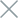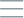• Home
• Tags
• Series
•# Local Search Algorithm in Java

The Local Search algorithm is a search technique in Java programming that focuses on optimizing solutions by searching within a vicinity of the current solution. Instead of searching the entire solution space, the algorithm concentrates on finding solutions in a smaller "neighborhood."

## How Local Search Algorithm Works

The algorithm starts from an initial solution and continually tries to improve it by searching for better solutions in the nearby vicinity. The algorithm iterates through nearby solutions and selects the best solution among them.

• Efficiency: The algorithm often operates faster in larger problem spaces by searching for nearby states instead of the entire space.
• Integration: Can be combined with other methods to enhance search performance.

• Local Optima: The algorithm might converge to a local optimal point without finding the global solution.

## Example and Explanation

A real-life example of the Local Search Algorithm is optimizing a traffic route. Let's see how this algorithm works:

``````import java.util.*;

public class LocalSearchExample {
static double evaluateSolution(double[] solution) {
// Function to evaluate the quality of a solution
// Lower value indicates a better solution
return 1.0 / (1.0 + solution + solution);
}

static double[] localSearch(double[] initialSolution, double stepSize, int maxIterations) {
double[] currentSolution = Arrays.copyOf(initialSolution, initialSolution.length);
double currentEvaluation = evaluateSolution(currentSolution);

for (int i = 0; i < maxIterations; i++) {
double[] nextSolution = Arrays.copyOf(currentSolution, currentSolution.length);
nextSolution += stepSize;
double nextEvaluation = evaluateSolution(nextSolution);

if (nextEvaluation < currentEvaluation) {
currentSolution = nextSolution;
currentEvaluation = nextEvaluation;
} else {
stepSize /= 2;
}
}

return currentSolution;
}
}
``````

In the above example, we use the Local Search Algorithm to optimize a numerical solution. The algorithm searches within a vicinity of the current solution by altering a fixed step and checks if the new solution is better. The result is that the algorithm progressively finds a better solution over time.